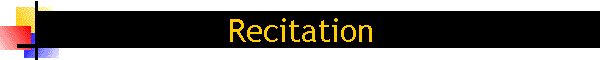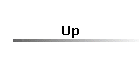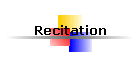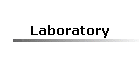Analytical Schedule of Recitation1st week Topics: Review of Electromagnetic Theory: Maxwell's equations in free space Constitutive relations Maxwell's equations in matter Boundary conditions Power and Energy Time Harmonic Electromagnetic Fields Electromagnetic Theory and Circuit Theory   Review:   Methodology: Techniques for the solution of problems:Analytical exact solutions of problems with nice geometryL, S, V, dl, ds, dv can be expressed in a coordinate system.Have a look on a mathematical handbook for a catalog of available coordinate systems.Other problems can be approximated or not solved at all using exact techniques.   Basic Points: Draw a diagram. Select appropriate coordinate system Write down an equation that give us the unknown quantity as function of known quantities. Solve the equation Plot given data and results Comment on your results   Examples:   Applications:   References: NetSeminar 2ndweek Topics: Electrical Properties of Matter Dielectrics Magnetics Linear - Homogeneous - Isotropic - Dispersive media The Materials in the presence of an AC field   The wave equation Solution of the wave equation   Review:   Methodology:   Basic Points:   Examples:   Applications:   Homework:   References: 3rd week Topics:   Methodology:   Basic Points:   Examples:   Applications:   Homework:   References: 4th week Topics:   Review:   Methodology:   Basic Points:   Examples:   Applications:   Homework:   References: 5th week Topics:   Review:   Methodology:   Basic Points:   Examples:   Applications:   Homework:   References: 6th week Topics:   Review:   Methodology:   Basic Points:   Examples:   Applications:   Homework:   References: 7th week Topics:   Review:   Methodology:   Basic Points:   Examples:   Applications:   Homework:   References: 8th week Topics:   Review:   Methodology:   Basic Points:   Examples:   Applications:   Homework:   References: 9th week Topics:   Review:   Methodology:   Basic Points:   Examples:   Applications:   Homework:   References: 10th week Topics:   Review:   Methodology:   Basic Points:   Examples:   Applications:   Homework:   References: 11th week Topics:   Review:   Methodology:   Basic Points:   Examples:   Applications:   Homework:  References: 12th week Topics:   Review:   Methodology:   Basic Points:   Examples:   Applications:   Homework:   References: 13th week Topics:   Review:   Methodology:   Basic Points:   Examples:   Applications:   Homework:   References: 14th week Topics:   Review:   Methodology:   Basic Points:   Examples:   Applications:   Homework:   References: 15th week Topics:   Review:   Methodology:   Basic Points:   Examples:   Applications:   Homework:   References: 16th week Final examination